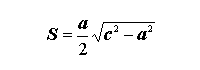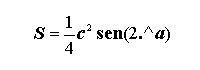Mathematics

Elements of a Triangle

 Show figure Calculate:   Area of the Triangle (S) Perimeter (p) Hypotenuse (c) Cathetus a Cathetus b Angle ^a Angle ^b 1- Cathetus a:2- Cathetus b: 3- Hypotenuse c:4- Angle ^a: 5- Angle ^b:To Memory 01 Memory 02 Memory 03 Memory 04 Memory 05 Memory 06 Replacing Adding Subtracting Multiplying Dividing
 Configurations Maximum of decimal places Unit of Measurement of Length: Impression Page Title (Optional) Comments to be printed (Optional)